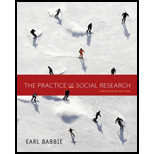Chapter 7, Problem 2RQE### The Practice of Social Research (M...

14th Edition
Earl R. Babbie
ISBN: 9781305104945

#### Solutions

Chapter
Section### The Practice of Social Research (M...

14th Edition
Earl R. Babbie
ISBN: 9781305104945
Textbook Problem

# Using Appendix B of this book, select a simple random sample of 10 numbers in the range of 1 to 9876. What is each step in the process?

Summary Introduction

Introduction

Step 1 is to number the list of random numbers

Step 2 is to select the number of digits, in this case it would be four

Step 3 is to create a plan for how to select numbers

Step 4 is to decide how to select 4 of the 5 digits

Step 5 is to select the numbers

6375, 2386, 1655, 3018, 9140, 6322, 1935, 1805, 5188, 1457

Explanation

(This question calls for a subjective answer. This is an example of an appropriate response.)

The first step in selecting random numbers is to arrange the list of numbers, in this case the list in appendix B is arranged randomly. The next step is to select the number of digits, and in this case the number is four, since the upper range of the sample is 9876...

### Still sussing out bartleby?

Check out a sample textbook solution.

See a sample solution

#### The Solution to Your Study Problems

Bartleby provides explanations to thousands of textbook problems written by our experts, many with advanced degrees!

Get Started

## Chapter 7 Solutions